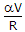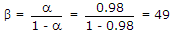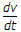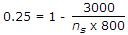# Electronics and Communication Engineering - Power Electronics

### Exercise :: Power Electronics - Section 1

26.

Current derating may be necessary when thyristors are operating in

 A. series B. parallel C. series or parallel D. none of the above

Explanation:

Since current does not divide equally, current derating is necessary.

27.

If duty cycle is a and the load is a resistance R, the rms value of output voltage is

 A. aV B. a V C. a2V D.Explanation:

RMS output voltage

VL = a V.

28.

For a BJT a = 0.98, then β =

 A. 0.02 B. 0.5 C. 4.9 D. 49

Explanation:29.

Assertion (A): Power transistor has lower switching time than SCR

Reason (R): Power transistor has greater switching time than SCR

 A. Both A and R are correct and R is correct explanation of A B. Both A and R correct but R is not correct explanation of A C. A is correct but R is wrong D. A is wrong but R is correct

Explanation:

Power transistor does not have highrating.

30.

A 3 kV circuit uses SCR of 800 V rating. If derating is 25%, the number of SCRs in series is

 A. 4 B. 5 C. 6 D. 8.